Courses
Courses for Kids
Free study material
Free LIVE classes
More

# Magic SquaresLIVE
Join Vedantu’s FREE Mastercalss

## The Popular Magic Squares

Magic Square is an interesting and very popular square that contains various different numbers arranged in such a way that the total sum of digits in each column, row and diagonal is the same.

And for this reason, this square puzzle is known as a magic square. Now you must be curious about how to make these magic squares and what is the formula of magic squares. So come let’s find the answers to all your questions in this article.

## What is a Magic Square?

Magic squares are an excellent mathematical wonder for kids. It is a big square which is made up of the same number of rows with different numbers. And in this, each line of the square that may be horizontal/vertical or even diagonal makes up the same sum and this sum is known as the “magic number”.

For example, The magic square given below has numbers ranging from 1 to 9 and just look at how all the numbers in each column, row and diagonal add up to the magic number 15.Magic Square

## What is the Formula for Magic Squares?

The magic square puzzle of the order $\mathrm{n}$ is a group of “$n{ }^{2}$” digits in a big square. And the magic square consists of the numbers from 1 to $n 2$. And these numbers that are in the rows, columns and diagonals will add up to the same sum or the "magic number". And this magic number is denoted by the letter $\mathrm{M}$ whose value completely depends on the value of $n$.

So the formula to find the value of a magic number is:

$M=\dfrac{n\left(n^2+1\right)}{2}$

## Tricks To Solve Magic Squares

As we got to know the formula for finding the magic number/sum is $\dfrac{n\left(n^2+1\right)}{2}$ so to find the magic square of order 3, the first step we'll substitute $n=3$ so that it'll be easy to form a magic square $3 \times 3$.

Now, $n=3$, the sum $=3(32+1)$

$\dfrac{3 \times 10}{2}=15 \text {. }$

So we will have to place the digits accordingly so that the numbers in all the rows, columns and diagonals will be equal to number 15.

## Trick for Magic Square of Order 3

Let’s assume “x” as the order of our magic square.

Let's assume "$x$" as the order of our magic square.

So here, $x=3$

Let's consider the second number "$y$" so that the product of $x$ and $y$ will be equal to the magic number/sum, i.e., 15.

So, $y=5\{x y=(3)(5)=15\}$

Now put the value of $y$ at the centre of the magic square and $x$ on its left. And the cell above $x$ should be $y-1$ as you can see below:Formula for Building Magic Square

Now according to this trick, magic squares of order 3 can be easily built.Magic Square of Order 3

### Magic Square 3 x 3

Let’s learn to make multiple magic squares of the same order by changing the numbers in different places from the magic square that we obtained above.

By taking the magic square $3 \times 3$ sum 15 that is shown below:Magic Square 3 x 3

Let us make another magic square of the above square:

$\left(n^2+1\right)=32+1=9+1=10$

Next step is to subtract each number from $(n^2+1)$, that is 10 .

So first row numbers will be:

$10-4=6$

$10-3=7$

$10-8=2$

Second row numbers will be:

$10-9=1$

$10-5=5$

$10-1=9$

Third row numbers will be:

$10-2=8$

$10-7=3$

$10-6=4$

Finally the new magic square that is formed is will be:The Newly Formed Magic Square

## Magic Squares Worksheet

1. Worksheet 1: Magic Squares for BeginnersMagic Squares Worksheet for Beginners

Ans: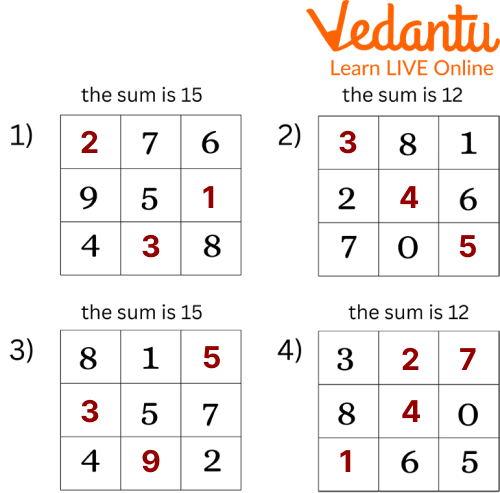Magic Squares Worksheet for Beginners

2. Worksheet 2: Magic Square Puzzles:Magic Square Puzzle Worksheet

Ans: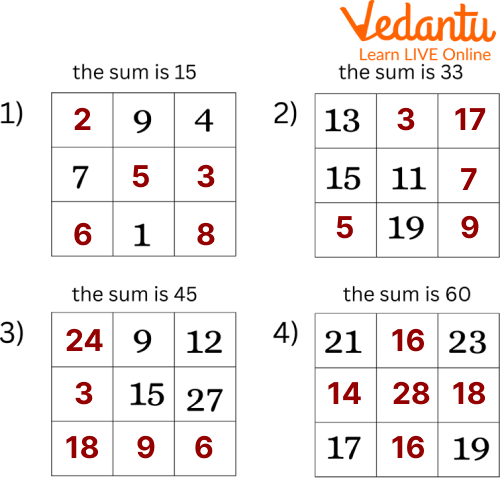Magic Square Puzzle Worksheet

## Practice Questions

Q 1. Fill the missing integrals in each of the magic squares and the magic number/sum is given.

1. The magic number is 21.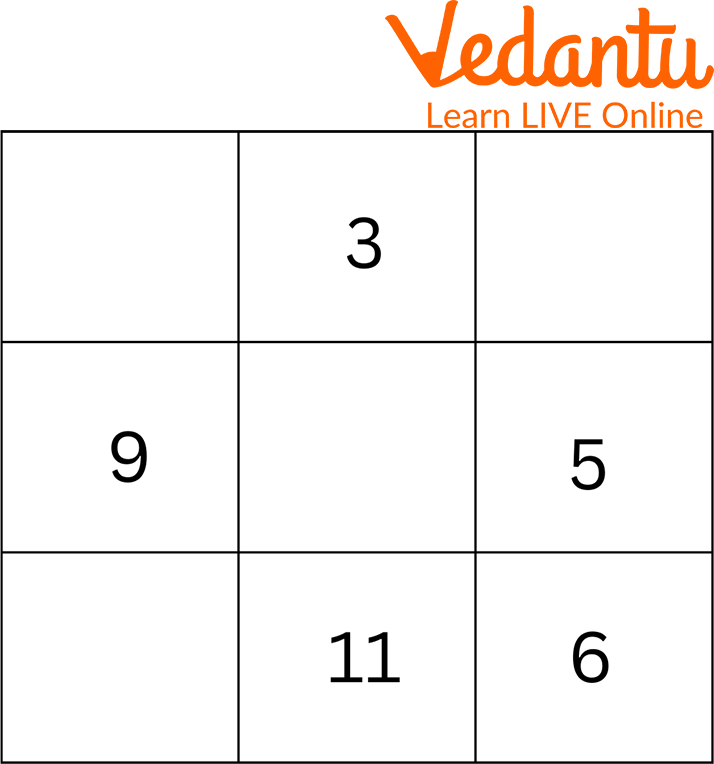Practice Question 1

1. The magic number is 36.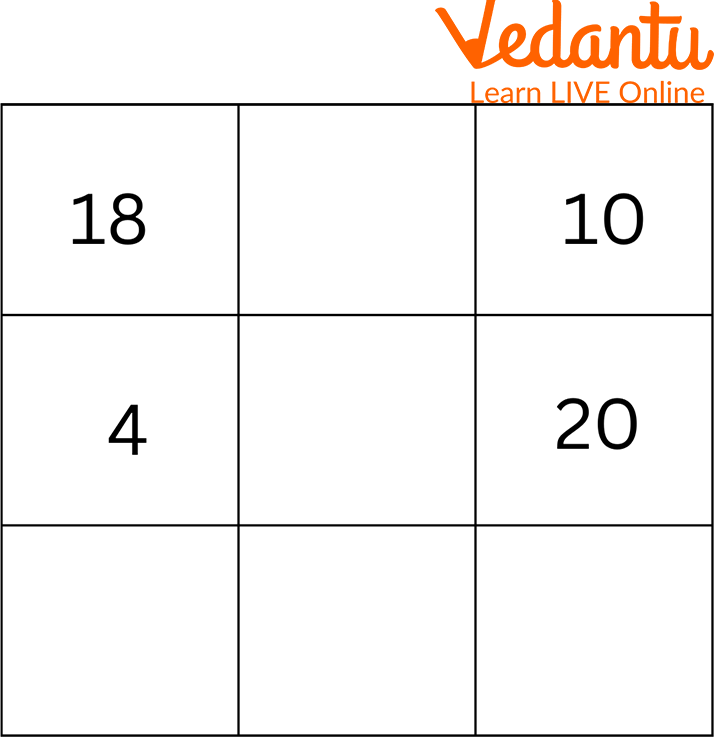Practice Question 2

1. The magic number is 27.Practice Question 3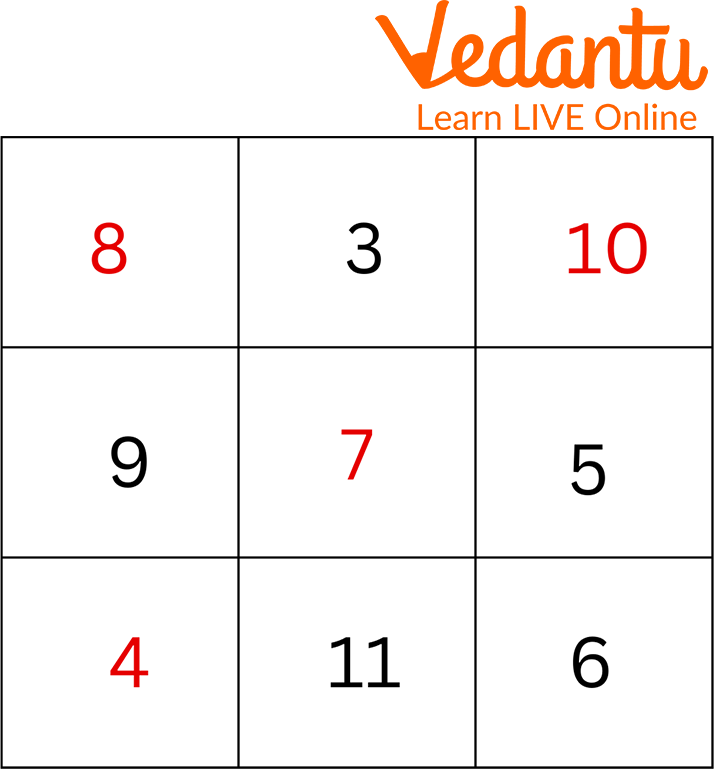Practice Question 1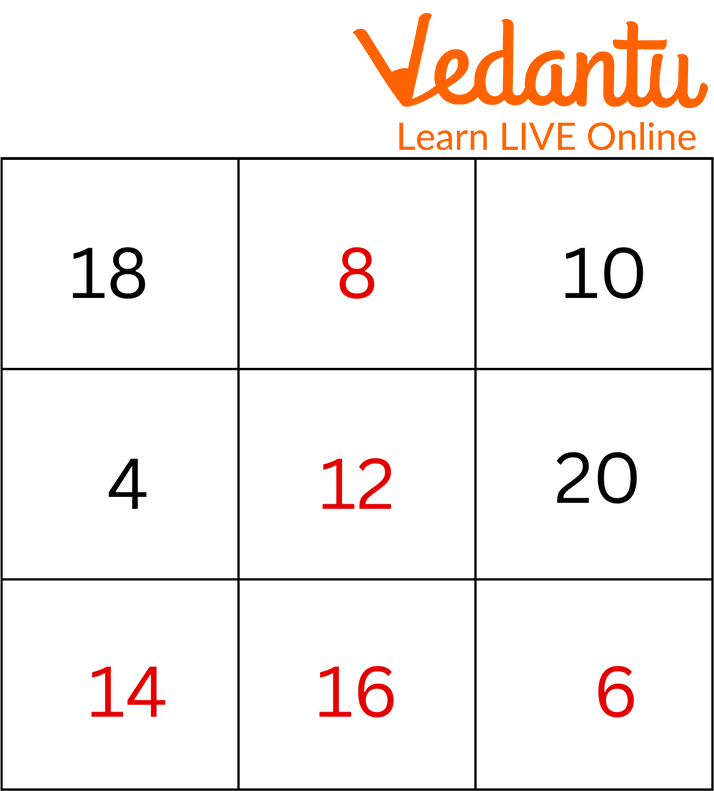Practice Question 2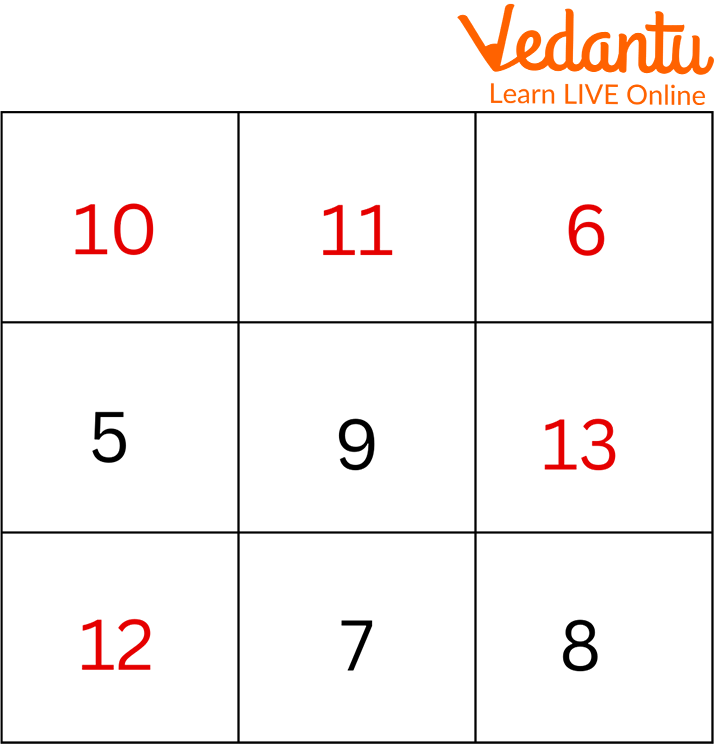Practice Question 3

## Summary

In this article we got to know about the magic squares. These magic square puzzles are a great way to learn mathematics calculations in an easy way for kids. They also help in developing the addition and subtraction skills and strategies for solving the magic square questions and make it more engaging and interesting. And after solving the given worksheets and practice questions it will be easier for you to find the missing numbers in a magic square and even build a new magic square.

Last updated date: 25th Sep 2023
Total views: 90.3k
Views today: 2.90k

## FAQs on Magic Squares

1. What is the history of a magic square?

History of the magic square is traced back as far as 2800B.C. to ancient China, the legend of Lo Shu tells us a story of a time when rivers were flooding and the river god sent a turtle to answer the people’s cry for help. And then a child noticed that a dotted pattern on a turtle’s back had a magic square, where each of the numbers in all the rows and columns added up to the sum 15.

2. When was the magic square made?

In the year 1694 and 1965, Yueki Ando gave different methods to create the magic squares and displayed the magic squares of order 3 to 30.

3. How big can a magic square be?

A magic square can be as small as $3 \times 3 \text { and as big as } 16 \times 16$ square.